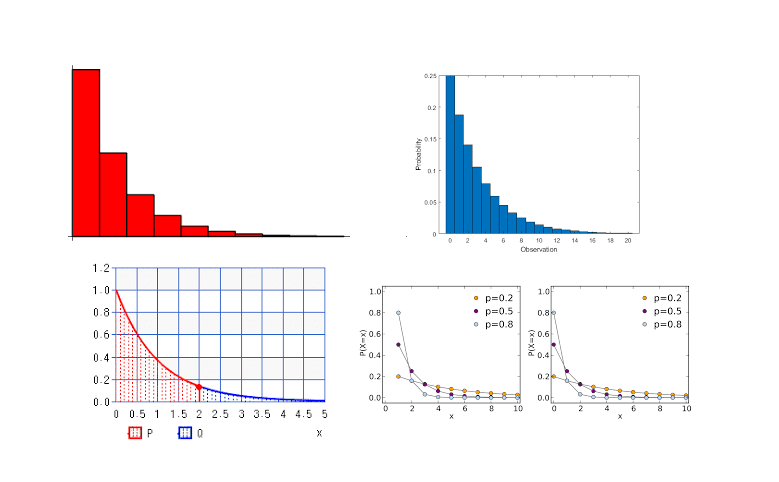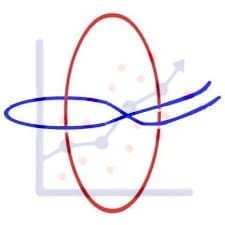Categories

# Geometry of Wilson’s Theorem

Wilson’s Theorem is a beautiful result from Number Theory. We created an animation on it! Linked it with Geometry and behold the beauty of mathematics that comes forth. Try it!

# Understand the problem

Wilson’s Theorem from Number Theory is a beautiful classical result. Enjoy this beautiful geometric proof of the classical result.

We used animations to bring the idea into life.

# Now watch the discussion video#### Kazi Abu Rousan

Cheenta Creative Team

Kazi is a student of Physics. He loves the relation between physics and mathematics. He is the creator of this beautiful video.

# Tutorial Problems… try these after watching the video.

1. Write down a rigorous proof of Wilson’s Theorem. 2. Is the converse of Wilson’s Theorem true? Can you prove it?

You may send solutions to support@cheenta.com. Though we usually look into internal students’ work, we will try to give you some feedback.

# Connected Program at Cheenta

Math Olympiad is the greatest and most challenging academic contest for school students. Brilliant school students from over 100 countries participate in it every year. Cheenta works with small groups of gifted students through an intense training program. It is a deeply personalized journey toward intellectual prowess and technical sophistication.

# Similar Problems

## Carpet Strategy in Geometry | Watch and Learn

Here is a video solution for a Problem based on Carpet Strategy in Geometry. This problem is helpful for Math Olympiad, ISI & CMI Entrance, and other math contests. Watch and Learn! Here goes the question… Suppose ABCD is a square and X is a point on BC such that...## Bijection Principle Problem | ISI Entrance TOMATO Obj 22

Here is a video solution for a Problem based on Bijection Principle. This is an Objective question 22 from TOMATO for ISI Entrance. Watch and Learn! Here goes the question… Given that: x+y+z=10, where x, y and z are natural numbers. How many such solutions are...## What is the Area of Quadrilateral? | AMC 12 2018 | Problem 13

Here is a video solution for a Problem based on finding the area of a quadrilateral. This question is from American Mathematics Competition, AMC 12, 2018. Watch and Learn! Here goes the question… Connect the centroids of the four triangles in a square. Can you find...## Solving Weird Equations using Inequality | TOMATO Problem 78

Here is a video solution for ISI Entrance Number Theory Problems based on solving weird equations using Inequality. Watch and Learn! Here goes the question… Solve: 2 \cos ^{2}\left(x^{3}+x\right)=2^{x}+2^{-x} We will recommend you to try the problem yourself. Done?...

## AM-GM Inequality Problem | ISI Entrance

Here is a video solution for ISI Entrance Number Theory Problems based on AM-GM Inequality Problem. Watch and Learn! Here goes the question… a, b, c, d are positive real numbers. Prove that: (1+a)(1+b)(1+c)(1+d) <= 16. We will recommend you to try the problem...

## Sum of 8 fourth powers | ISI Entrance Problem

Here is a video solution for ISI Entrance Number Theory Problems based on Sum of 8 fourth powers. Watch and Learn! Can you show that the sum of 8 fourth powers of integers never adds up to 1993? How can you solve this fourth-degree diophantine equation? Let’s...

## ISI MStat Entrance 2020 Problems and Solutions

Problems and Solutions of ISI MStat Entrance 2020 of Indian Statistical Institute.

## ISI Entrance 2020 Problems and Solutions – B.Stat & B.Math

Problems and Solutions of ISI BStat and BMath Entrance 2020 of Indian Statistical Institute.## Testing of Hypothesis | ISI MStat 2016 PSB Problem 9

This is a problem from the ISI MStat Entrance Examination,2016 making us realize the beautiful connection between exponential and geometric distribution and a smooth application of Central Limit Theorem.## ISI MStat PSB 2006 Problem 8 | Bernoullian Beauty

This is a very simple and regular sample problem from ISI MStat PSB 2009 Problem 8. It It is based on testing the nature of the mean of Exponential distribution. Give it a Try it !## By Ashani Dasgupta

Founder Director at Cheenta
Pursuing Ph.D. in Mathematics from University of Wisconsin Milwaukee
Research Interest - Geometric Topology

This site uses Akismet to reduce spam. Learn how your comment data is processed.